Age Group: 3-6 years

Addition and Subtraction are the foundation of Advanced Maths and a solid understanding of the same is essential for your child to build strong mathematical skills in coming years. While addition comes a little easy to understand, many children often struggle with subtraction. I would present here four different ways which would help children grasp the concept of subtraction.

# Familiarize The Child With The Scenarios Where Subtraction Applies

Before starting with subtraction, introduce your child to the vocabulary/signs like minus, difference, takeaway, equals etc. and the real world problem statements where subtraction applies. Consider these examples:

Example 1: Maitri has 5 apples. She gives 2 of them to her friend Radha. How many apples are still with Maitri?

Example 2: There are 4 members in John’s family. Two of them went out. How many are at home?

Example 3: Mary had 20 dollars in her pocket. She purchased an ice-cream for 1 dollar. How much money is she left with?

The easiest way for a kindergarten or first grader to imagine subtraction is as something taken away or a loss or decrease of quantity. Give them sufficient examples with a very small subtrahend (e.g., involving a decrease of one or two) such that they can use their counting experience to give the answer and more time is spent in understanding the problem scenarios. Now consider various subtraction methods.

# Method 1: Subtraction As Take Away

This is the most intuitive method. Though we do not have the provision of negative numbers in this, however kids at kindergarten age do not require that.

Example 4: Give 4 cookies to your child. Now ask him to share 2 of them with you or a sibling. Let him count the remaining cookies. (In case your child is not willing to share the cookies, ask him to eat two of them and count the remaining :-) ).

Once the child understands the concept with real objects it would be easy for him/her to use a pencil and paper to draw the objects. Consider the same cookie example: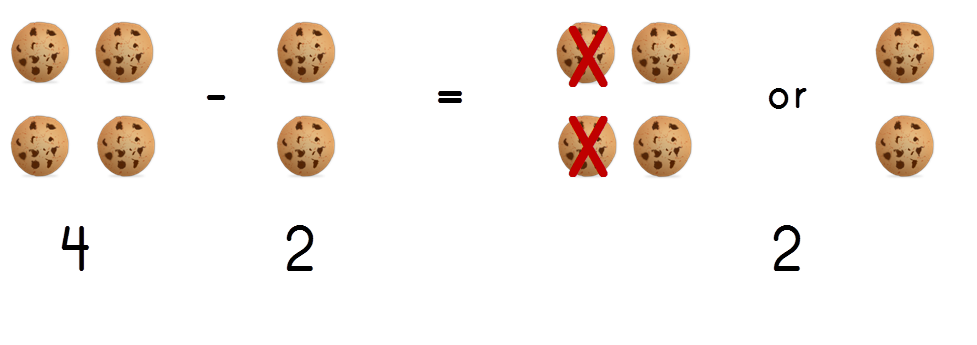After a little practice children can straight away carry out the subtraction by using standing lines or circles. Make as many circles/standing lines as the total number of objects available (or minuend). Then strike through or cross the objects to be subtracted (subtrahend). Count the remaining standing lines/circles; this should give the remaining items or difference.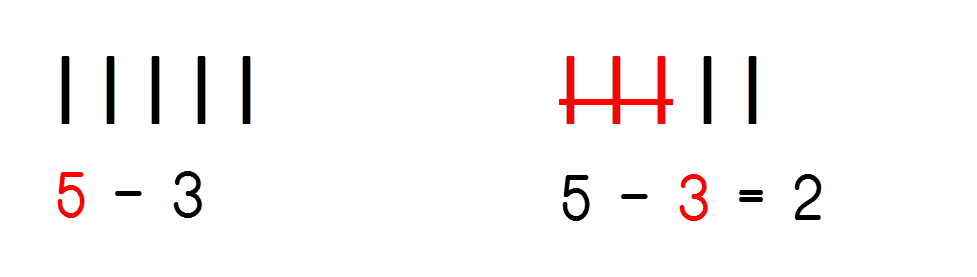Gradually kids can practice to subtract on fingers.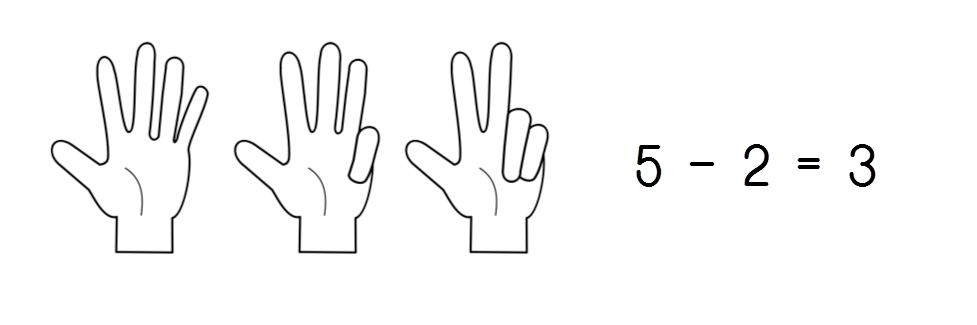# Method 2: Subtraction Using Number Line (Counting Back)

Draw a number line. Make a circle at the minuend which is the starting point. Now count back hopping on the line as many times as the subtrahend. The number on the line where you reach is the difference.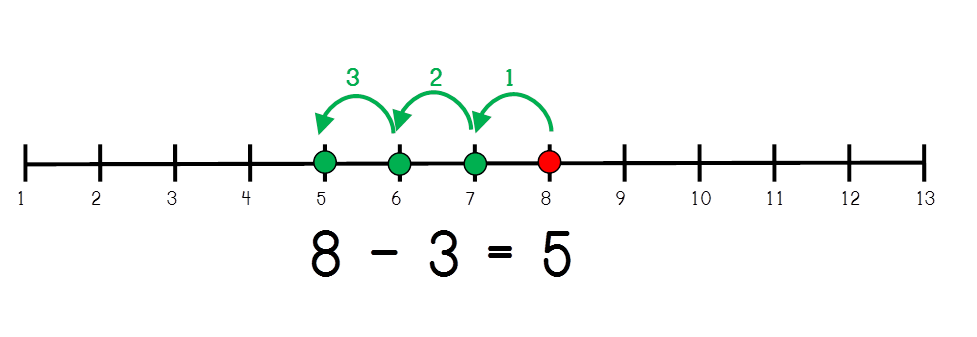# Method 3: Subtraction Using Number Line (Finding Difference)

This is similar to Method 2 however instead of counting back we find the difference between the two numbers. Make a circle at the minuend and subtrahend on the number line. Now start counting from the subtrahend till you reach minuend. The final count is the difference.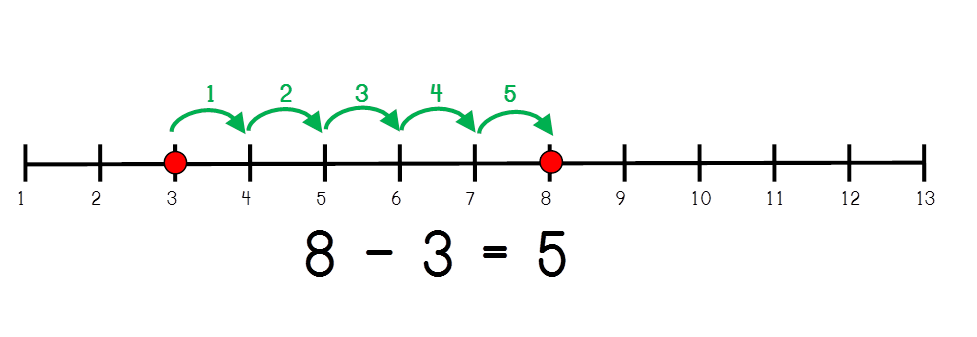You can find some practice worksheets for subtraction using number line here.

# Method 4: Subtraction Using Reverse Counting

The concept is same as of Method 2 however instead of using a number line we just do backward counting as many times as the subtrahend. For example, consider 8-3:With all these different ways to learn subtraction, your child would surely have happy learning experience.